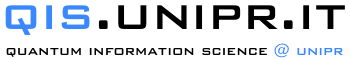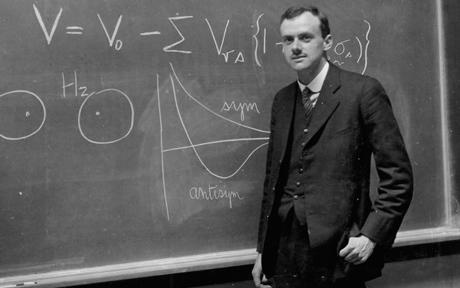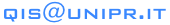Other seminars:

Related courses:

 2018 Lecture series on Quantum Information (1) Introduction to Quantum Mechanics (8h), prof. Sandro Wimberger Abstract: A general introduction to quantum mechanics will be given, based on experiments with single phtons, with applications in cryptography. In the second part, the mathematical formalism will be introduced, that is necessary to develop and understand the quantum theory. The concept of entanglement between different subsystems will be introduced. Explicit examples of quantum states will help understanding the lectures' contents. (2) Introduction to Quantum Information Processing (8h), prof. Stefano Carretta Abstract: In this part of the course, the concept of qubit will be introduced, basic logical operations will be discussed, together with the basic principles of quantum computation. Moreover, the concept of entanglement will be discussed, as well as its most simple applications. Finally, quantum simulators will be introduced. (3) Quantum Algorithms and Protocols (8h), prof. Michele Amoretti Abstract: In this part of the course, some fundamental quantum algorithms will be introduced, such as Shor's factorization algorithm and Grover's unstructured search algorithm. Moreover, a short tutorial on IBM quantum computing system will be provided. Finally, the concept of Quantum Internet will be introduced, with reference to some important quantum protocols. Past Seminars Sandro Wimberger and Stefano Carretta Introduction into Quantum Mechanics (QM) 18, 25 Jan. and 1 Feb. 2017, 2:30 pm @ DII Sandro Wimberger Quantum cryptography: A pedestrian introduction 16 Feb. 2016, 2:30 pm @ DII